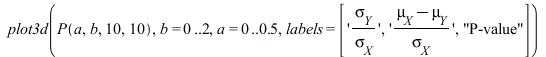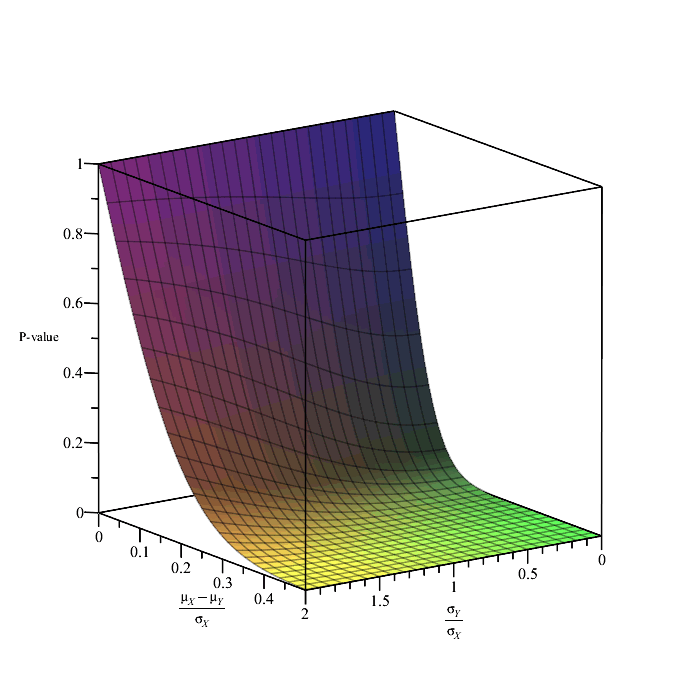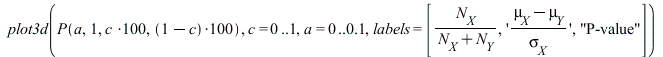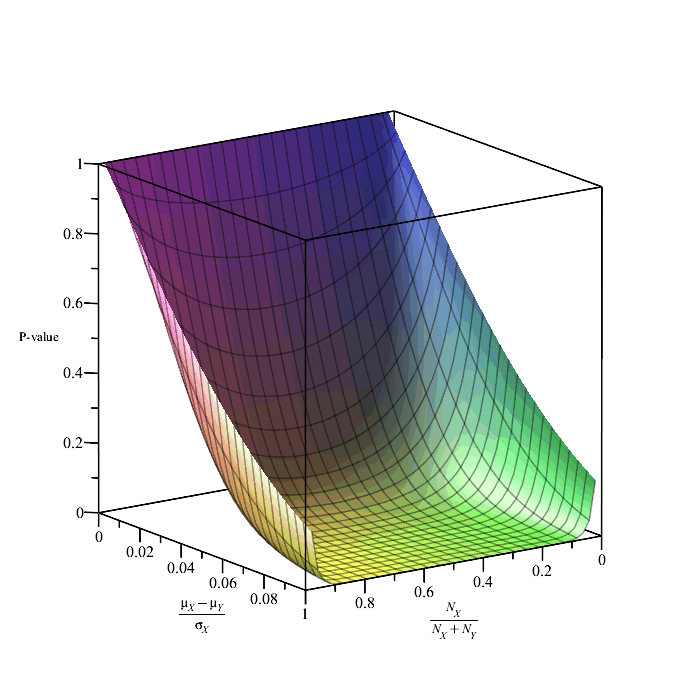Parametric Exploration of the Mann-Whitney U Test

Abraham Nunes MD PhD MBA

Dalhousie University, Halifax, Nova Scotia, Canada

The Binormal ROC Model and the U-Statistic

Recall that the CDF of a normal distribution is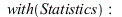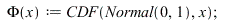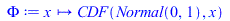(1.1)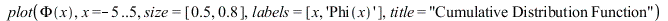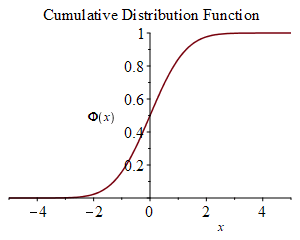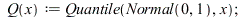(1.2)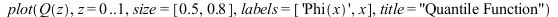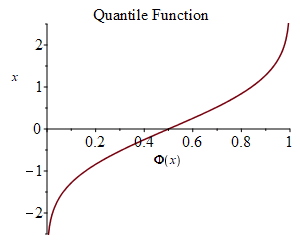Assuming that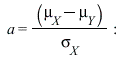and thatwe can compute the ROC curve for two normal distributions as follows: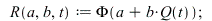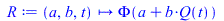(1.3)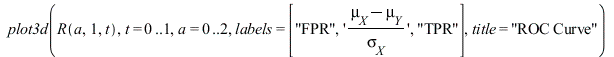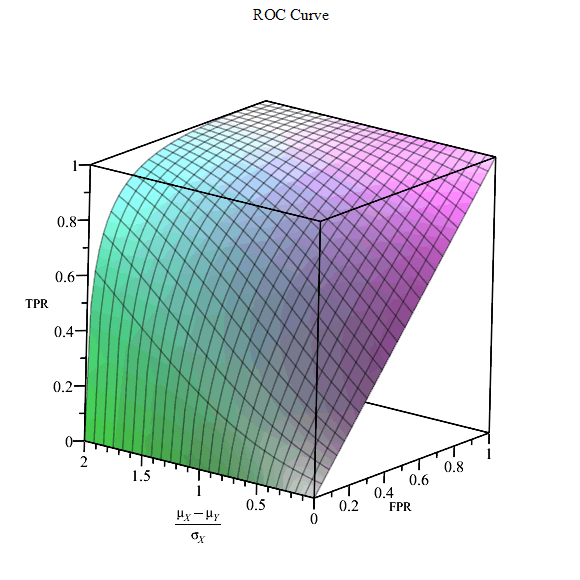The area under the ROC curve (AUC) can be computed as follows: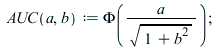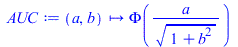(1.4)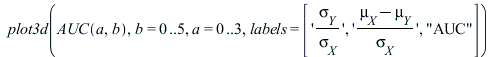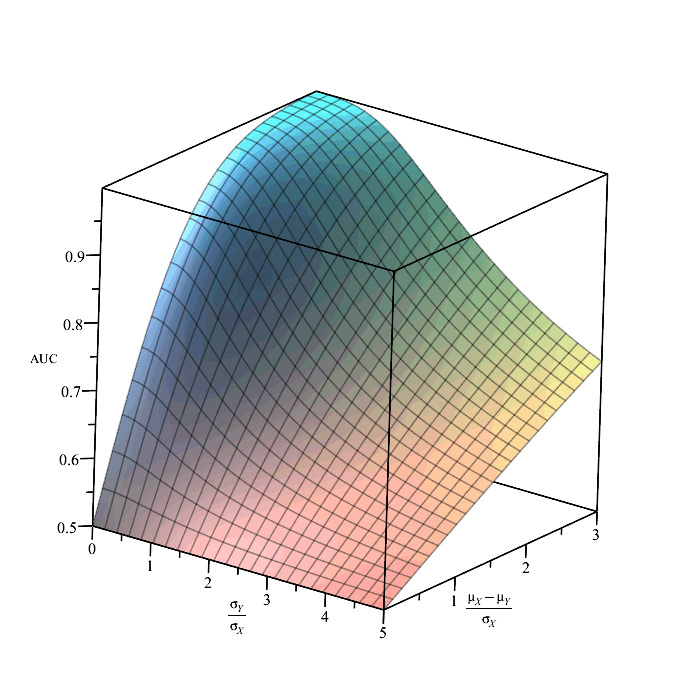The U-statistic for the binormal model with sample sizes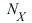and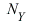can be computed from the AUC as follows: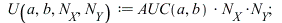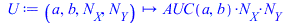(1.5)

Computing P-Values from the U-Statistic

At large samples, the U-statistic is normally distributed with mean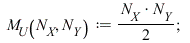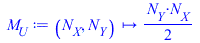(2.1)

and the standard deviation is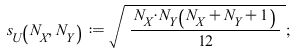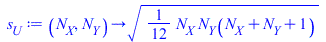(2.2)

We can therefore compute a Z-score: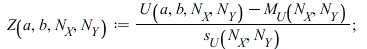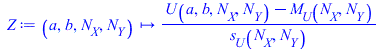(2.3)

A two-tailed p-value is thus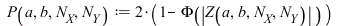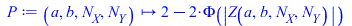(2.4)